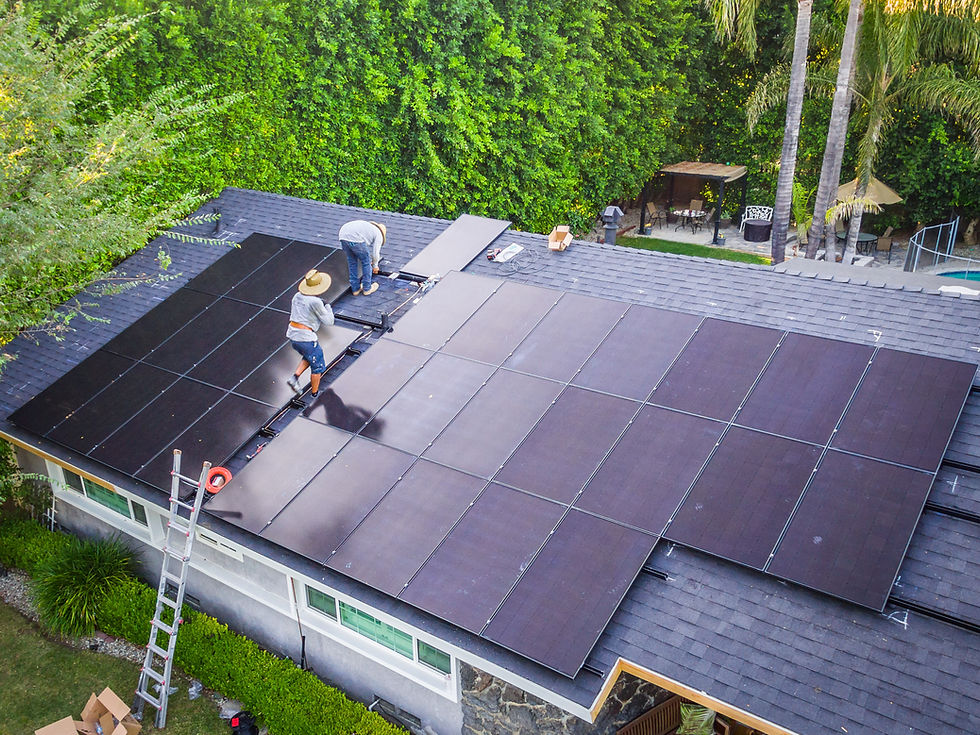top of page# (MACRS) Commercial Only# MACRS Explained

The Modified Accelerated Cost Recovery System (MACRS) is a tax depreciation system that allows commercial PV owners to depreciate the value of their solar energy investment over a period of time, thereby reducing their taxable income and increasing their return on investment.

In general, MACRS allows businesses to recover the cost of an asset through annual depreciation deductions over a predetermined recovery period.

The MACRS depreciation deduction for a solar energy system can be calculated using the following formula:

Depreciation = (Cost basis - Salvage value) x Depreciation percentage

The cost basis is the total cost of the solar energy system, including installation, engineering, and design costs, excluding any state or federal incentives or rebates received. The salvage value is the estimated value of the system at the end of its useful life, which is typically assumed to be zero for solar energy systems.

The depreciation percentage is determined by the recovery period and the depreciation method selected. For solar energy systems, the percentage is a 200% declining balance with a switch to straight-line depreciation in the first year that provides the maximum depreciation deduction in the early years of the project.

To calculate the depreciation deduction for a solar energy system, let's assume a cost basis of \$500,000 and a 21% tax rate:

Year 1: Depreciation = (\$500,000 - \$0) x 40% x 21% = \$42,000

Year 2: Depreciation = (\$500,000 - \$42,000) x 40% x 21% = \$33,956

Year 3: Depreciation = (\$500,000 - \$75,956) x 40% x 21% = \$26,973

Year 4: Depreciation = (\$500,000 - \$102,929) x 20% x 21% = \$11,017

Year 5: Depreciation = (\$500,000 - \$113,946) x 20% x 21% = \$8,978

In this example, the solar energy system owner would be able to claim a total depreciation deduction of \$122,924 over the first five years of the project, reducing their taxable income and increasing their return on investment.

Overall, MACRS provides a valuable incentive for commercial PV owners to invest in solar energy systems, helping to accelerate the rate of return on their investment and making solar energy a more attractive option for businesses.

bottom of page